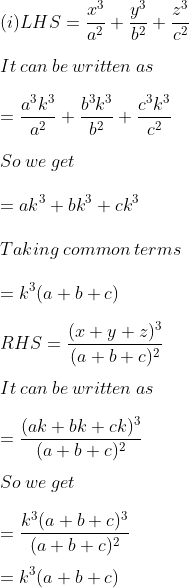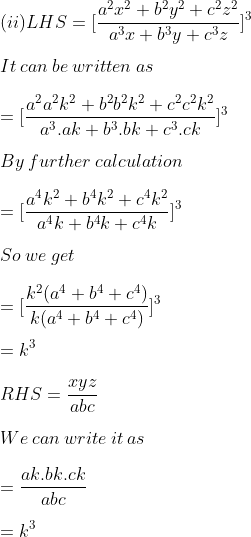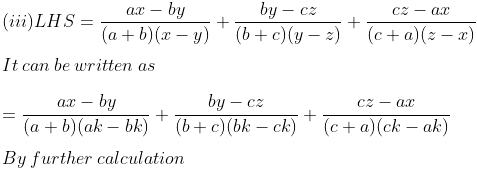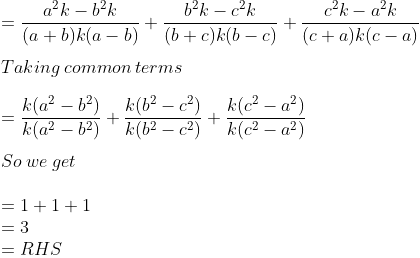Guru

# If x/a = y/b = z/c then prove that (i)x³/a²+ y³/b²+z³/c²=(x+y+z)³/(a+b+c)² (ii) [a²x²+b²y²+c²z²/a³x+b³y+c³z] ³=xyz/abc (iii)(ax-by) /(a+b)(x-y) +(by-cz) /(b+c)(y-z) +(cz-ax) /(c+a) (z-x) =3

• 0

exam oriented an important question from ML aggarwal, class 10th, chapter 7, ratio and proportion, Avichal publication

It is given that x/a = y/b = z/c

Then prove that

(i)x³/a²+ y³/b²+z³/c²=(x+y+z)³/(a+b+c)²

(ii) [a²x²+b²y²+c²z²/a³x+b³y+c³z] ³=xyz/abc

(iii)(ax-by) /(a+b)(x-y) +(by-cz) /(b+c)(y-z) +(cz-ax) /(c+a) (z-x) =3

Question no. 16, exercise 7. 2

Share

1. Solution:

It is given that

x/a = y/b = z/c

We can write it as

x = ak, y = bk and z = ckTherefore, LHS = RHS.Therefore, LHS = RHS.• 0